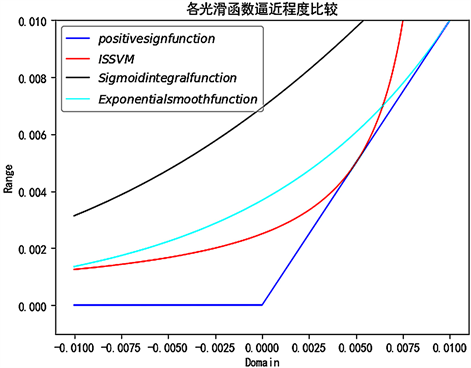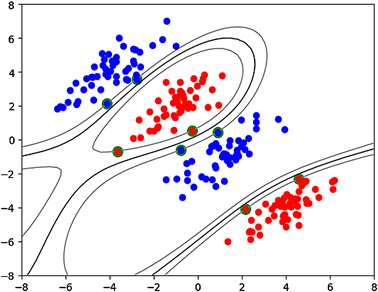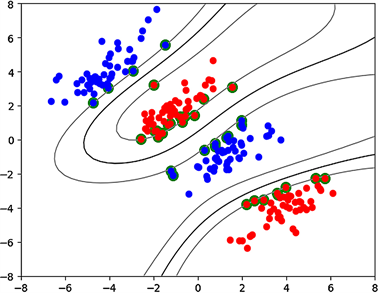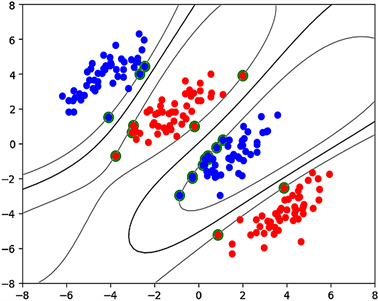﻿ 反比例光滑支撑向量机

反比例光滑支撑向量机Inverse Proportional Smooth Support Vector Machine

Abstract: In order to improve the classified performance of smooth support vector machine model, a new smoothed function inverse proportional function is presented, and smoothing technique is used to overcome the non-differentiability of support vector machine model. Through rigorous demonstration and analysis in mathematical theory, the properties of the given smoothing function and the convergence of the inverse proportional smoothing support vector machine (ISSVM) model based on the smoothing function are proved. The experimental results show that the inverse proportional smoothing support vector machine is superior to the polynomial smoothing support vector machine in classified performance.

1. 引言

2001年Lee等人通过对支撑向量机的深入研究引入光滑的概念，使用了sigmoid积分函数p(x,α)对无约束的支撑向量机模型SVM  进行光滑化，得出了分类性能较好的光滑支撑向量机SSVM 。继而使用Newton-Armijo算法对SSVM进行求解，结果展现出了SSVM比标准SVM具有更好的分类性能和较高的计算效率，从此光滑函数作为SSVM模型的核心引起了人们的广泛关注，并开辟了支撑向量机的一个新的研究方向。

2005年文献  提出了两个多项式光滑函数，同时对无约束的支撑向量机模型进行光滑化处理，引出了PSSVM模型。可以证明，PSSVM比SSVM有更好的分类性能。之后文献  提出了三阶样条光滑函数，从而引出了新的支撑向量机的TSSVM模型。通过分析TSSVM模型的收敛性，并通过数据实验可以说明TSSVM分类器的效果要更优。2008年，文献  提出了六阶光滑函数，此光滑函数逼近正号函数的精度比三阶样条光滑函数更是高了一个数量级。2014年，吴青等人在此基础上提出指数光滑支持向量分类机  的模型，证明了其收敛性并通过数值实验表明了指数光滑支持向量机比多项式光滑支持向量机在分类性能上更有优势。

2. 反比例光滑函数

$i\left(x,k\right)=\left\{\begin{array}{l}x,\text{\hspace{0.17em}}\text{\hspace{0.17em}}x>\frac{1}{10k}\\ \frac{1}{10k\left(2-10kx\right)},\text{\hspace{0.17em}}\text{\hspace{0.17em}}x\le \frac{1}{10k}\end{array}$

1) $i\left(x,k\right)$ 关于x一阶光滑。

2) 当 $x\to -\infty$$k>0$ 时， $i\left(x,k\right)\to 0$

${i}^{\prime }\left(x,k\right)=\left\{\begin{array}{l}1,\text{\hspace{0.17em}}\text{\hspace{0.17em}}x>\frac{1}{10k}\\ \frac{1}{{\left(2-10kx\right)}^{2}},\text{\hspace{0.17em}}\text{\hspace{0.17em}}x\le \frac{1}{10k}\end{array}$

$\underset{x\to \frac{1}{10k}-}{\mathrm{lim}}\frac{1}{{\left(2-10kx\right)}^{2}}=1$

${i}^{″}\left(x,k\right)=\left\{\begin{array}{l}0,\text{\hspace{0.17em}}\text{\hspace{0.17em}}x>\frac{1}{10k}\\ \frac{20k}{{\left(2-10kx\right)}^{3}},\text{\hspace{0.17em}}\text{\hspace{0.17em}}x\le \frac{1}{10k}\end{array}$

$x\to -\infty$ 并且 $k>0$ 时，对 $i\left(x,k\right)$ 求极限，即得

$\underset{x\to -\infty }{\mathrm{lim}}\frac{1}{10k\left(2-10kx\right)}=0$

1) $i\left(x,k\right)\ge {x}_{+}$

2) 对于 $x\in \left(-\infty ,+\infty \right)$，当 $k>0$ 时，有

${i}^{2}\left(x,k\right)-{x}_{+}^{2}\le 0.00131105\frac{1}{{k}^{2}}$

${f}_{1}\left(x,k\right)=i\left(x,k\right)-{x}_{+}=\frac{1}{10k\left(2-10kx\right)}-x$

${f}_{1}\left(x,k\right)$ 求导得

${{f}^{\prime }}_{1}\left(x,k\right)=\frac{1}{{\left(2-10kx\right)}^{2}}-1<0\text{\hspace{0.17em}}\text{\hspace{0.17em}}\text{\hspace{0.17em}}\left(0\le x\le \frac{1}{10k}\right)$

${f}_{1}\left(x,k\right)$$\left(0,\frac{1}{10k}\right)$ 内单调递减，又因 ${f}_{1}\left(x,k\right)$$x=\frac{1}{10k}$ 处取得最小值0，因此

${f}_{1}\left(x,k\right)=i\left(x,k\right)-{x}_{+}\ge 0$

${f}_{2}\left(x,k\right)=i\left(x,k\right)-0=\frac{1}{10k\left(2-10kx\right)}>0$

$x\ge \frac{1}{10k}$ 时，结论(2)显然成立。当 $0 时， ${x}_{+}=x$，于是对

${g}_{1}\left(x,k\right)={i}^{2}\left(x,k\right)-{x}^{2}=\frac{1}{100{k}^{2}{\left(2-10kx\right)}^{2}}-{x}^{2}$

${{g}^{\prime }}_{1}\left(x,k\right)=\frac{1}{5k{\left(2-10kx\right)}^{3}}-2x$

${x}_{0}=0.020312\frac{1}{k}$

${g}_{1}\left({x}_{0},k\right)=0.00131105\frac{1}{{k}^{2}}$

${g}_{2}\left(x,k\right)={i}^{2}\left(x,k\right)=\frac{1}{100{k}^{2}{\left(2-10kx\right)}^{2}}$

$\left(-\infty ,0\right]$ 上单调递增，且其最大值

${g}_{2}\left(0,k\right)=\frac{1}{400{k}^{2}}=0.0025\frac{1}{{k}^{2}}$

${i}^{2}\left(x,k\right)-{x}_{+}^{2}\le 0.00131105\frac{1}{{k}^{2}}<0.0188\frac{1}{{k}^{2}}$Figure 1. The approximating degree of smooth functions

3. ISSVM模型及其收敛性

$\begin{array}{c}f\left(x\right)=\text{sign}\left[\underset{i=1}{\overset{m}{\sum }}\text{ }\text{ }{y}_{i}{\alpha }_{i}^{*}\left(\phi \left({x}_{i}\right),\phi \left(x\right)\right)+{b}^{*}\right]\\ =\text{sign}\left[\underset{i=1}{\overset{m}{\sum }}\text{ }\text{ }{y}_{i}{\alpha }_{i}^{*}K\left({x}_{i},x\right)+{b}^{*}\right]\end{array}$ (1)

${b}^{*}=\frac{1}{m}\underset{i=1}{\overset{m}{\sum }}\left({y}_{i}-\underset{i=1}{\overset{m}{\sum }}\text{ }\text{ }{y}_{i}{\alpha }_{j}^{*}\left(\phi \left({x}_{j}\right),\phi \left({x}_{i}\right)\right)\right)$ (2)

$M={\left({x}_{1},{x}_{2},\cdots ,{x}_{m}\right)}^{\text{T}}$

${x}_{i}=\left({x}_{i}{}_{1},{x}_{i}{}_{2},\cdots ,{x}_{i}{}_{n}\right)\text{\hspace{0.17em}}\left(i=1,2,\cdots ,m\right)，$

$e=\text{ones}\left(m,1\right)$$D=\text{diag}\left({y}_{1},{y}_{2},\cdots ,{y}_{m}\right)$$H=DK\left(M,{M}^{\text{T}}\right)D$

$\left\{\begin{array}{l}\underset{\alpha ,b}{\mathrm{min}}\text{\hspace{0.17em}}\frac{1}{2}{\alpha }^{\text{T}}H\alpha +C{e}^{\text{T}}\xi \\ \text{s}\text{.t}\text{.}\text{\hspace{0.17em}}\text{\hspace{0.17em}}D\left(K\left(M,{M}^{\text{T}}\right)D\alpha +eb\right)-e+\xi \ge 0,\xi \ge 0\end{array}$ (3)

$\left\{\begin{array}{l}\underset{\alpha ,b}{\mathrm{min}}\text{\hspace{0.17em}}\frac{1}{2}\left({\alpha }^{\text{T}}\alpha +{b}^{2}\right)+\frac{c}{2}{\xi }^{\text{T}}\xi \\ \text{s}\text{.t}.\text{\hspace{0.17em}}\text{\hspace{0.17em}}D\left(K\left(M,{M}^{\text{T}}\right)D\alpha +eb\right)-e+\xi \ge 0,\xi \ge 0\end{array}$ (4)

$\xi ={\left(e-D\left(K\left(M,{M}^{\text{T}}\right)D\alpha +eb\right)\right)}_{+}$

$\underset{\alpha ,b}{\mathrm{min}}\frac{1}{2}\left({\alpha }^{\text{T}}\alpha +{b}^{2}\right)+\frac{c}{2}{‖{\left(e-D\left(K\left(M,{M}^{\text{T}}\right)D\alpha +eb\right)\right)}_{+}‖}^{2}$ (5)

$\underset{\alpha ,b}{\mathrm{min}}\frac{1}{2}\left({\alpha }^{\text{T}}\alpha +{b}^{2}\right)+\frac{c}{2}{‖i\left(e-D\left(K\left(M,{M}^{\text{T}}\right)D\alpha +eb\right),k\right)‖}_{2}^{2}$ (6)

$\omega =\alpha ,\gamma =-b,A=K\left(M,{M}^{\text{T}}\right)D$，则上式可化为

$\underset{\omega ,\gamma }{\mathrm{min}}\text{\hspace{0.17em}}\frac{1}{2}\left({\omega }^{\text{T}}\omega +{\gamma }^{2}\right)+\frac{c}{2}{‖i\left(e-D\left(A\omega -e\gamma \right),k\right)‖}_{2}^{2}$

${f}_{+}\left(x\right):{ℝ}^{n}\to ℝ$

${f}_{+}\left(x\right)=\frac{1}{2}{‖{\left(Ax-b\right)}_{+}‖}_{2}^{2}+\frac{1}{2}{‖x‖}_{2}^{2}$

${f}_{i}\left(x,k\right):{ℝ}^{n}×ℕ\to ℝ$

${f}_{i}\left(x,k\right)=\frac{1}{2}{‖i\left(Ax-b,k\right)‖}_{2}^{2}+\frac{1}{2}{‖x‖}_{2}^{2}$

1) ${f}_{+}\left(x\right)$${f}_{i}\left(x,k\right)$ 都是强凸函数。

2) 优化函数 $\underset{x}{\mathrm{min}}{f}_{+}\left(x\right)$ 存在唯一解 ${x}^{*}$，优化函数 $\underset{x}{\mathrm{min}}{f}_{i}\left(x,k\right)$ 存在唯一解 ${\left({x}^{*}\right)}^{k}$

3) 对于任意的 $k\ge 1$，有

${‖{\left({x}^{*}\right)}^{k}-{x}^{*}‖}^{2}\le \frac{0.00131105\text{m}}{2{k}^{2}}$

4) ${x}^{*}$${\left({x}^{*}\right)}^{k}$ 满足

$\underset{k\to \infty }{\mathrm{lim}}{\left({x}^{*}\right)}^{k}={x}^{*}$

${L}_{v}\left({f}_{i}\left(x,k\right)\right)\subseteq {L}_{v}\left({f}_{+}\left(x\right)\right)\subseteq \left\{x:{‖x‖}^{2}\le 2v\right\}\left(v\ge 0\right)$

5) 我们不妨假设 ${x}^{*}$${\left({x}^{*}\right)}^{k}$ 分别是 $\underset{x}{\mathrm{min}}{f}_{+}\left(x\right)$$\underset{x}{\mathrm{min}}{f}_{i}\left(x,k\right)$ 的唯一解，则有

${f}_{+}\left({\left({x}^{*}\right)}^{k}\right)-{f}_{+}\left({x}^{*}\right)\ge \nabla {f}_{+}\left({x}^{*}\right)\left({\left({x}^{*}\right)}^{k}-{x}^{*}\right)+\frac{1}{2}{‖{\left({x}^{*}\right)}^{k}-{x}^{*}‖}_{2}^{2}=\frac{1}{2}{‖{\left({x}^{*}\right)}^{k}-{x}^{*}‖}_{2}^{2}$

${f}_{i}\left({x}^{*},k\right)-{f}_{i}\left({\left({x}^{*}\right)}^{k},k\right)\ge \nabla {f}_{i}\left({\left({x}^{*}\right)}^{k},k\right)\left({x}^{*}-{\left({x}^{*}\right)}^{k}\right)+\frac{1}{2}{‖{\left({x}^{*}\right)}^{k}-{x}^{*}‖}_{2}^{2}=\frac{1}{2}{‖{\left({x}^{*}\right)}^{k}-{x}^{*}‖}_{2}^{2}$

$\begin{array}{c}{‖{\left({x}^{*}\right)}^{k}-{x}^{*}‖}_{2}^{2}\le \left({f}_{i}\left({x}^{*},k\right)-{f}_{+}\left({x}^{*}\right)\right)-\left({f}_{i}\left({\left({x}^{*}\right)}^{k},k\right)-{f}_{+}\left({\left({x}^{*}\right)}^{k}\right)\right)\\ \le {f}_{i}\left({x}^{*},k\right)-{f}_{+}\left({x}^{*}\right)=\frac{1}{2}{‖i\left(A{x}^{*}-b,k\right)‖}_{2}^{2}-\frac{1}{2}{‖{\left(A{x}^{*}-b\right)}_{+}‖}_{2}^{2}\end{array}$

${‖{\left({x}^{*}\right)}^{k}-{x}^{*}‖}^{2}\le \frac{0.00131105\text{m}}{2{k}^{2}}$

$\underset{k\to \infty }{\mathrm{lim}}{‖{\left({x}^{*}\right)}^{k}-{x}^{*}‖}^{2}\le \underset{k\to \infty }{\mathrm{lim}}\frac{0.00131105m}{2{k}^{2}}=0$

$\underset{k\to \infty }{\mathrm{lim}}{\left({x}^{*}\right)}^{k}={x}^{*}$

4. 求解ISSVM模型的BFGS算法

${H}^{0}=I,\text{\hspace{0.17em}}\text{\hspace{0.17em}}\left(\left({\omega }^{0}\right),{\gamma }^{0}\right)={p}^{0}\in {ℝ}^{n+1},\text{\hspace{0.17em}}\epsilon ={10}^{-8},\text{\hspace{0.17em}}{\alpha }^{0}=I,\text{\hspace{0.17em}}i=0,$

${F}^{i}=F\left({p}^{i},k\right),\text{\hspace{0.17em}}{g}^{i}=\nabla F\left({p}^{i},k\right),$

${‖{g}^{i}‖}_{2}^{2}\le \epsilon$ 或迭代次数达到最大，那么迭代停止，并取 $\left({\left({\omega }^{i}\right)}^{\text{T}},{\gamma }^{i}\right)={p}^{i}$ 为ISSVM模型的最优参数解；

${s}^{i}=-{\alpha }^{i}{H}^{i}{g}^{i}$

${F}^{i+1}=F\left({p}^{i+1},k\right),\text{\hspace{0.17em}}{g}^{i+1}=\nabla F\left({p}^{i+1},k\right),\text{\hspace{0.17em}}{y}^{i}={g}^{i+1}-{g}^{i}$

${H}^{i+1}=\left(I-\frac{{s}^{i}{\left({y}^{i}\right)}^{\text{T}}}{{\left({s}^{i}\right)}^{\text{T}}{y}^{i}}\right){H}^{i}\left(I-\frac{{y}^{i}{\left({s}^{i}\right)}^{\text{T}}}{{\left({s}^{i}\right)}^{\text{T}}{y}^{i}}\right)+\frac{{s}^{i}{\left({s}^{i}\right)}^{\text{T}}}{{\left({s}^{i}\right)}^{\text{T}}{y}^{i}}$

5. 数值实验Table 1. Experiment of NDC data setFigure 2. The result (1) of classificationFigure 3. The result (2) of classificationFigure 4. The result (3) of classificationFigure 5. The result (4) of classificationTable 2. Experiment of checkerboard data set

6. 结语

 Vapnik, V.N. (1995) The Nature of Statistical Learning Theory. Spring Verlag, New York, 1-299.
https://doi.org/10.1007/978-1-4757-2440-0_1

 Lee, Y.J. and Mangasarian, O.L. (2001) SSVM: A Smooth Support Vector Machine for Classification. Computational Optimization and Applications, 20, 5-22.
https://doi.org/10.1023/A:1011215321374

 袁玉波, 严杰, 徐成贤. 多项式光滑的支撑向量机[J]. 计算机学报, 2005, 28(1): 9-17.

 Yuan, Y.B., Fan, W.G. and Pu, D.M. (2007) Spline Function Smooth Support Vector for Classification. Journal of Industrial and Management Optimization, 3, 529-542.
https://doi.org/10.3934/jimo.2007.3.529

 黄仁泰, 熊金志. 一个支持向量机的新光滑函数及其性能分析[J]. 计算机工程与应用, 2008, 44(18): 64-65.

 吴青, 王婉, 王玲芝. 指数光滑支持向量分类机[J]. 西安邮电大学学报, 2014, 19(4): 9-14.

 Song, J.L., Gan, X.B. and Song, D.X. (2004) Nonlinear Global Optimization Based on Bisection Method. Journal of Information and Computational Science, 1, 229-233.

 Yuan, Y.B. and Byrd, R. (1995) Non-Quasi-Newton Updates for Unconstrained Optimization. Journal of Computing Mathematics, 13, 95-107.

 Yuan, Y.B. (1991) A Modified BFGS Algorithm for Uncontrained Optimization. IMA Journal of Nu-merical Analysis, 11, 325-332.
https://doi.org/10.1093/imanum/11.3.325

 吴青, 赵雄. 一类新样条光滑支持向量机[J]. 西安邮电大学学报, 2013, 18(6): 68-74.

 Stone, M. (1974) Cross-Validatory Choice and Assessment of Statistical Prediction. Journal of the Royal Statistical Society, 36, 111-147.
https://doi.org/10.1111/j.2517-6161.1974.tb00994.x

 Mangasarian, O.L. and Musicant, D.R. (1999) Succes-sive Over-Relaxation for Support Vector Machines. IEEE Transactions on Neural Networks, 10, 1032-1037.
https://doi.org/10.1109/72.788643

Top